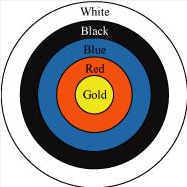# NCERT Solutions for Class 10 Maths Chapter 12 Areas Related to Circles Exercise 12.1

In this page we have NCERT Solutions for Class 10 Maths Chapter 12 Areas Related to Circles for EXERCISE 12.1 . Hope you like them and do not forget to like , social share and comment at the end of the page.
Question 1) The radii of the two circles are 19cm and 9 cm respectively. Find the radius of the circle which has circumference equal to the sum of the circumferences of the two circles.
Solution 1:
According to the question
Circumference of Ist circle =  2π(19)  =38 π cm
Circumference of 2nd  circle =  2π(9)  =18 π cm
Let r be the radius of the new circle
Circumference of New  circle =  2π(r)  =2 πr cm
Now
2πr=38π+18π
Or r =28 cm
Question 2) The radii of two circles are 8 cm and 6 cm respectively. Find the radius of the circle having area equal to the sum of the areas of the two circles.
Solution 2:
According to the question
Area of Ist circle =πr2=π(64)=64π
Area of 2nd circle =πr2=π(36)=36π
Let  r the radius of new circle
Area of New circle=πr2
Now as per question
πr2= 64 π+36 π
r=10cm
Question 3. The below depicts an archery target marked with its five scoring regions from the centre outwards as Gold, Red, Blue,Black and White. The diameter of the region representing Gold score is 21 cm and each of the other bands is 10.5 cm wide. Find the area of each of the five scoring regions.Solution:
Diameter of Gold Circle is given as =21 cm
Area of Gold region is given by
= πr2= (22/7) (10.5)2= 346.5 cm 2
Now since each band  is 10.5 cm wide
Radius of red ring= 10.5+ 10.5=21 cm
Now Radius of Red ring is given as
= π(21)2  - π(10.5)2  = 1386-346.5=1039.5 cm2
Radius of Blue ring =21 +10.5=31.5 cm
Now Radius of Blue ring is given as
= π(31.5)2  - π(21)2  = 3118.5-1386=1732.5 cm2
Radius of Black ring =31.5 +10.5=42 cm
Now Radius of Black ering is given as
= π(42)2  - π(31.5)2  = 5544-3118.5=2425.5 cm2
Radius of White ring =42 +10.5=52.5 cm
Now Radius of Black ering is given as
= π(52.5)2  - π(42)2  = 8662.5-5544=3118.5 cm2
Question 4)  The wheels of a car are of diameter 80 cm each. How many complete revolutions does each wheel make in 10 minutes when the car is travelling at a speed of 66 km per hour?

Solution:
Diameter of wheel is given as =80 cm
So radius of the wheel=40 cm
So circumference of wheel =2πr= 2(22/7)(40)= (1760/7)cm
So in 1 revolution, Car travels the distance  (1760/7)cm
Now Speed of the Car= 66Km/h
=(66X1000)/60   meter/minute
=1100 meter/minute
=110000 cm/minute
This mean in simple term
Distance travelled in 1 min =  110000 cm
Distance travelled in 10 min=1100000  cm
So Total number of revolutions =( 1100000)/(1760/7)
=4375
Question 5. Tick the correct answer in the following and justify your choice : If the perimeter and the area of a circle are numerically equal, then the radius of the circle is
(A) 2 units
(B) π units
(C) 4 units
(D) 7 units
Solution:
Area of Circle  = πr2
Circumference of Circle =2πr
According to the question
πr2 = 2πr
r=2 unitsGo back to Class 10 Main Page using below links

### Practice Question

Question 1 What is $1 - \sqrt {3}$ ?
A) Non terminating repeating
B) Non terminating non repeating
C) Terminating
D) None of the above
Question 2 The volume of the largest right circular cone that can be cut out from a cube of edge 4.2 cm is?
A) 19.4 cm3
B) 12 cm3
C) 78.6 cm3
D) 58.2 cm3
Question 3 The sum of the first three terms of an AP is 33. If the product of the first and the third term exceeds the second term by 29, the AP is ?
A) 2 ,21,11
B) 1,10,19
C) -1 ,8,17
D) 2 ,11,20